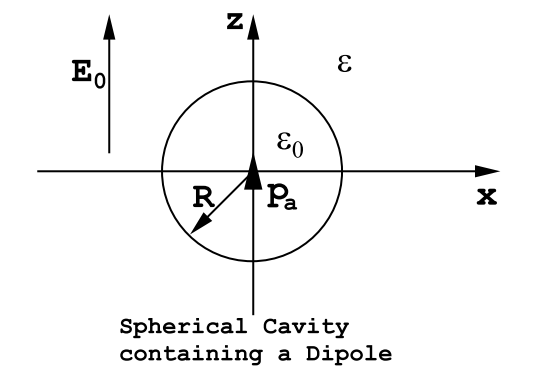$$\require{cancel}$$

# 3.4: The Field Energy as Minimum

Consider a group of conductors embedded in a dielectric medium as shown in Figure (3.3.13). Let the actual potential distribution be V corresponding to the electric field $$\overrightarrow{\mathrm{E}}=-\operatorname{grad}(\mathrm{V})$$; the potential function V satisfies LaPlace’s equation, ∇2V = 0, and it satisfies the boundary conditions. Now consider a second potential distribution, Ve, that is not the correct one: $$\mathrm{V}_{\mathrm{e}}=\mathrm{V}+\delta \mathrm{V}$$, where $$\delta \mathrm{V}=0$$ on the conductors. This situation might arise, for example, if one tried to guess the potential distribution given the potential on each of the conductors. The field energy calculated using the wrong potential function Ve would be

$\mathrm{U}_{\mathrm{E}}^{1}=\int \int \int_{S p a c e}(\mathrm{d} \mathrm{Vol}) \frac{\epsilon}{2}(\overrightarrow{\mathrm{E}}+\delta \overrightarrow{\mathrm{E}}) \cdot(\overrightarrow{\mathrm{E}}+\delta \overrightarrow{\mathrm{E}}), \nonumber$

where $$\overrightarrow{\mathrm{E}}=-\operatorname{grad}(\mathrm{V})$$ and $$\delta \mathrm{E}=-g r a d(\delta \mathrm{V})$$. Upon multiplying out the factors under the integral sign one obtains

$\mathrm{U}_{\mathrm{E}}^{1}=\int \int \int_{S p a c e}(\mathrm{dVol})\left(\frac{\epsilon \mathrm{E}^{2}}{2}+\epsilon \overrightarrow{\mathrm{E}} \cdot \delta \overrightarrow{\mathrm{E}}+\frac{\epsilon}{2}(\delta \overrightarrow{\mathrm{E}})^{2}\right). \label{3.70}$

But

$\operatorname{div}(\overrightarrow{\mathrm{D}} \delta \mathrm{V})=\delta \mathrm{V} \operatorname{div}(\overrightarrow{\mathrm{D}})+\overrightarrow{\mathrm{D}} \cdot \operatorname{grad}(\delta \mathrm{V})=\rho_{f}(\delta \mathrm{V})-\overrightarrow{\mathrm{D}} \cdot \delta \overrightarrow{\mathrm{E}}. \nonumber$

If $$\operatorname{div}(\overrightarrow{\mathrm{D}} \delta \mathrm{V})$$ is integrated over all space the result is

$\int \int \int_{S p a c e}(\mathrm{dVol}) d i v(\overrightarrow{\mathrm{D}} \delta \mathrm{V})=\int \int_{S u r f a c e} \overrightarrow{\mathrm{d} \mathrm{S}} \cdot \overrightarrow{\mathrm{D}} \delta \mathrm{V}=\int \int \int_{S p a c e}(\mathrm{d} \mathrm{Vol}) \rho_{f} \delta \mathrm{V}-\int \int \int_{S p a c e}(\mathrm{dVol}) \overrightarrow{\mathrm{D}} \cdot \delta \overrightarrow{\mathrm{V}}. \label{3.71}$

The surface integral in Equation (\ref{3.71}) goes to zero for the usual reasons as the surface S becomes infinitely large. Namely, very far from any sources |$$\vec D$$| goes to zero at least as fast as 1/R2 and the surface area increases like R2 so that the surface integral contribution must vanish providing that the product $$|\overrightarrow{\mathrm{D}}| \delta \mathrm{V}$$ goes to zero at least as fast as 1/R3 , ie. $$\delta$$V must go to zero at least as fast as 1/R. Also by hypothesis the charge density, ρf , vanishes everywhere except on the surfaces of the conductors where $$\delta$$V = 0, by hypothesis. Consequently, Equation (\ref{3.71}) gives the result

$\int \int \int_{S p a c e}(\mathrm{dVol}) \overrightarrow{\mathrm{D}} \cdot \delta \overrightarrow{\mathrm{E}}=\int \int \int_{S p a c e}(\mathrm{dVol}) \epsilon \overrightarrow{\mathrm{E}} \cdot \delta \overrightarrow{\mathrm{E}}=0. \nonumber$

It follows from this and from Equation (\ref{3.70}) that the incorrect energy $$U_{E}^{1}$$ exceeds the correct energy UE, where

$\mathrm{U}_{\mathrm{E}}=\int \int \int_{S_{\mathrm{P} n} \alpha_{\mathrm{e}}}(\mathrm{d} \mathrm{Vol}) \frac{\epsilon}{2} \mathrm{E}^{2}, \nonumber$

by a positive definite amount:

$\delta \mathrm{U}_{\mathrm{E}}=\mathrm{U}_{\mathrm{E}}^{1}-\mathrm{U}_{\mathrm{E}}=\int \int \int_{S_{\text {pace}}}(\mathrm{dVol}) \frac{\epsilon}{2}(\delta \overrightarrow{\mathrm{E}})^{2}. \label{3.72}$

This demonstrates that the electric field energy is a minimum for the correct field distribution. This fact can be made the basis for an approximate method for solving electrostatic field problems: one guesses at the form of the potential using a reasonable function that contains a number of adjustable constants a,b,c etc. These constants are adjusted so as to obtain the minimum electrostatic energy. The solution so obtained represents the given functional form that most closely approximates the exact solution. This method has been illustrated in the last part of Chpt.(19) of the Feynman Lectures on Physics, Vol.(II), using a cylindrical capacitor as an example.Figure $$\PageIndex{18}$$: A point dipole pa is located at the center of an empty spherical cavity of radius R cut out of an otherwise homogeneous dielectric material characterized by a dielectric constant $$\epsilon$$. The electric field far from the cavity is E0 Volts/m, and is uniform and directed along the z-axis.